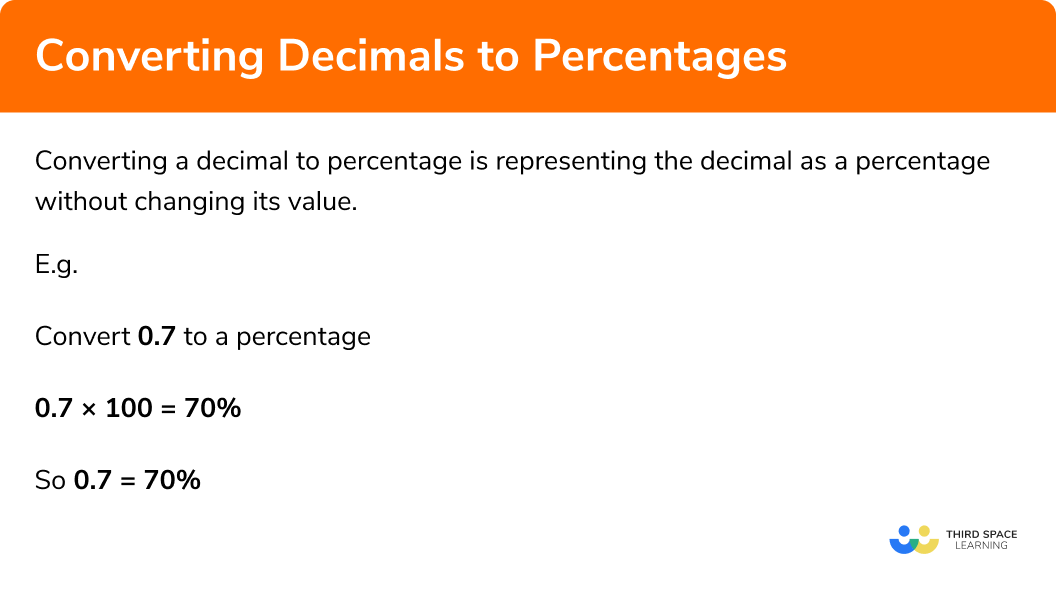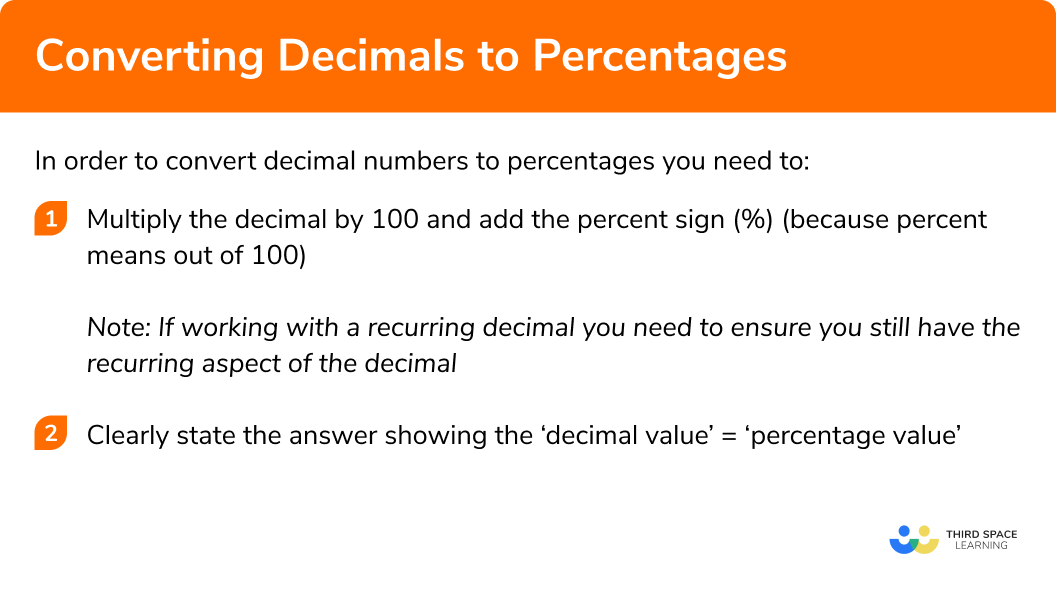GCSE Maths Number FDP Converting FDP

Decimal To Percentage

# Decimal To Percentage

Here we will learn about converting decimals to percentages.

There are also decimals to percentages worksheets based on Edexcel, AQA and OCR exam questions, along with further guidance on where to go next if you’re still stuck.

## What is converting decimals to percentages?

Converting a decimal to percentage is representing the decimal as a percentage without changing its value.

E.g.

\begin{aligned} &0.25=25\%\\\\ &0.45=45\%\\\\ &0.33333…=33.\dot{3}\%\\\\ &0.8=80\% \end{aligned}

### What is converting decimals to percentages?## How to convert decimals to percentages

In order to convert decimal numbers to percentages you need to:

1. Multiply the decimal by \pmb{100} and add the percent sign (%) (because percent means out of \pmb{100}).
Note: If working with a recurring decimal you need to ensure you still have the recurring aspect of the decimal.
2. Clearly state the answer showing the ‘decimal value’ = ‘percentage value’.

### Explain how to convert decimal numbers to percentages in 2 stepsTo learn how to convert percentages to decimals check out:

Step by step guide: Percentage to Decimal

## Converting decimals to percentages examples

### Example 1: converting a decimal to a percentage

Convert 0.7 to a percentage.

1.  Multiply the decimal by a hundred and add the percentage sign (%).

$0.7\times100=70\%$

The 7 has moved two places to the left. The decimal point does not move.

2Clearly state the answer showing the ‘decimal’ = ‘percentage’.

$0.7=70\%$

### Example 2: converting a decimal to a percentage

Convert 0.625 to a percentage.

\begin{aligned} &0.625\times100\\\\ &62.5\% \end{aligned}

$0.625 =62.5\%$

### Example 3: converting a decimal to a percentage (where the decimal is greater than 1)

Convert 1.23 to a percentage.

$1.23\times100=123\%$

$1.23=123\%$

### Example 4: converting a decimal to a percentage (involving thousandths)

Convert 0.006 to a percentage.

$0.006\times100=0.6\%$

$0.006=0.6\%$

### Example 5: converting a recurring decimal to a percentage

Convert 0.\dot{2} to a percentage.

$0.\dot{2} \times 100$

For this step you need to remember that the 2 is recurring; this means it is repeated infinitely.

0.\dot{2}=0.222222222222…

So when you multiply the recurring decimal by 100 you still have the recurring 2.

Therefore

$0.\dot{2}\times100= 22.\dot{2}$

$0.\dot{2}= 22.\dot{2}\%$

### Example 6: converting an improper fraction to a percentage

Convert 0.\dot{1}4285\dot{7} to a percentage.

$0.\dot{1}4285\dot{7} \times 100$

For this step you need to remember that the 142857 is recurring; this means it is repeated infinitely.

$0.\dot{1}4285\dot{7}=0.142857142857142857142857…$

So,

$0.\dot{1}4285\dot{7} \times 100 =14.2857142857142857142857…$

Therefore

\begin{aligned} & 0.\dot{1}4285\dot{7} \times 100 =14.\dot{2}8571\dot{4} \\\\ &14.\dot{2}8571\dot{4} \% \end{aligned}

$0.\dot{1}4285\dot{7} =14.\dot{2}8571\dot{4} \%$

### Calculator skills

If you are allowed to use a calculator for the questions, you can perform the operation in one calculation.

E.g.

Convert 1.057 to a percentage.

1. Enter the decimal on the calculator and multiply by \pmb{100}.
2. Press = (this will normally give you the answer in fraction form).
3. Press the \pmb{S ⇔ D} button to convert a decimal.
4. This means \pmb{ 1.057= 105.7\% }.

### Common misconceptions

• Mistakes with multiplication of \pmb{100}

Often mistakes are made when multiplying a value by 100 by moving the digits the incorrect number of decimal places.

• Not adding a percentage sign

Percentages must end in a percentage sign (%)
E.g.
50 is not a percentage but 50\% is.

• Not noticing a recurring decimal

Sometimes a recurring decimal is not immediately obvious.
E.g.
\frac{1}{7}=0.142857142857142857..

Therefore,
\frac{1}{7}= 0.\dot{1}4285\dot{7}

Decimal to percentage is part of our series of lessons to support revision on comparing fractions, decimals and percentages. You may find it helpful to start with the main comparing fractions, decimals and percentages lesson for a summary of what to expect, or use the step by step guides below for further detail on individual topics. Other lessons in this series include:

### Practice decimal to percentage questions

1. Convert 0.01 to a percentage

1\%10\%0.1\%0.01\%0.01 has been correctly multiplied by 100.

2. Convert 0.4 to a percentage

40\%4\%0.4\%400.4 has been correctly multiplied by 100.

3. Convert 1.1 to a percentage

11\%1.1\%110110\%1.1 has been correctly multiplied by 100.

4. Convert 0.006 to a percentage

0.60.06\%6\%0.6\%0.006 has been correctly multiplied by 100.

5. Convert 30.05 to a percentage

30.05\%3005\%3005305\%30.05 has been correctly multiplied by 100.

6. Convert 0.\dot{4} to a percentage

44.\dot{4}\%44\%44.4\%0.\dot{4}\%44.\dot{4}\% has been correctly multiplied by 100.

### Decimal to percentage GCSE questions

1. Convert each of the following decimals to percentages:

a) 0.09

b) 0.25

c) 0.9

d) 0.007

e) 6.6

(5 marks)

a) 9\%

(1)

b) 25\%

(1)

c) 90\%

(1)

d) 0.7\%

(1)

e) 660\%

(1)

2. Convert each of the following decimals to percentages:

a) 0.31

b) 0.887

c) 0.7\dot{3}

(4 marks)

a) 31\%

(1)

b) 88.7\%

(1)

c) 73.33333…\% or 73.\dot{3}

(1)

3.  Match the decimals to the percentage.

(5 marks)

0.41=41\%

(1)

0.5=50\%

(1)

1.7=170\%

(1)

2.6=260\%

(1)

0.003=0.3\%

(1)

4. Represent 0.\dot{5} as a percentage.

You must show your working out at each stage.

(2 marks)

0.\dot{5}\times100 or 55.\dot{5} seen

(1)

55.\dot{5}\%

(1)

## Learning checklist

You have now learned how to:

• Convert a decimal to a percentage
• Convert a recurring decimal to a percentage

## Still stuck?

Prepare your KS4 students for maths GCSEs success with Third Space Learning. Weekly online one to one GCSE maths revision lessons delivered by expert maths tutors.

Find out more about our GCSE maths tuition programme.